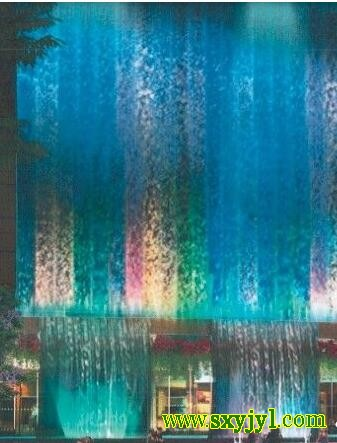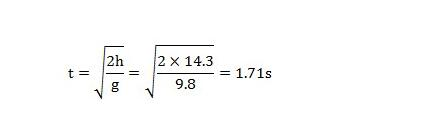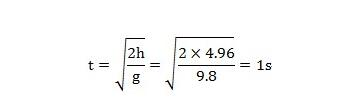﻿﻿ 人造瀑布设计计算源景园林-花园设计技术|论文源景园林-花园设计文库源景园林-花园设计陕西源景园林有限公司官网-西安屋顶绿化设计-庭院绿化-屋顶花园-陕西园林景观设计公司# 人造瀑布设计计算发布日期：2019-02-25  浏览次数：322

瀑布如上图示，由三层14.2米位置跌至一层0m，再由0m位置跌至地下一层 —4.96m处，三层位置瀑布沿玻璃幕墙面跌水，一层瀑布为普通悬挂式瀑布，两侧宽3.6m，中间宽7.2m 瀑布水量计算 瀑布跌落时间 瀑布体积 V= 长×宽×瀑布厚度×安全系数=14.2 ×19.4 ×0.003瀑布如上图示，由三层14.2米位置跌至一层0m，再由0m位置跌至地下一层 —4.96m处，三层位置瀑布沿玻璃幕墙面跌水，一层瀑布为普通悬挂式瀑布，两侧宽3.6m，中间宽7.2mV= 长×宽×瀑布厚度×安全系数=14.2 ×19.4 ×0.003 ×1.05=0.87m3

Q=V/t=0.87 ÷1.71=0.5m3/s

q=1/2Q=1/2 ×0.5=0.25m3/sV1=3.6 ×4.96 ×0.01 ×1.05=0.1875m3
V2=3.6 ×4.96 ×0.01 ×1.05=0.1875m3
V3=7.2 ×4.96 ×0.01 ×1.05=0.375m3
q1=q2=0.1875m3/s
q3=0.375m3/s

[ 文库搜索 ]  [ 加入收藏 ]  [ 告诉好友 ]  [ 打印本文 ]  [ 违规举报 ]  [ 关闭窗口 ]

﻿

（申请交换链接）

#### 客服中心